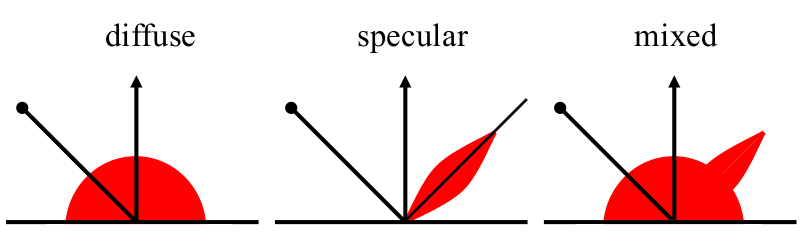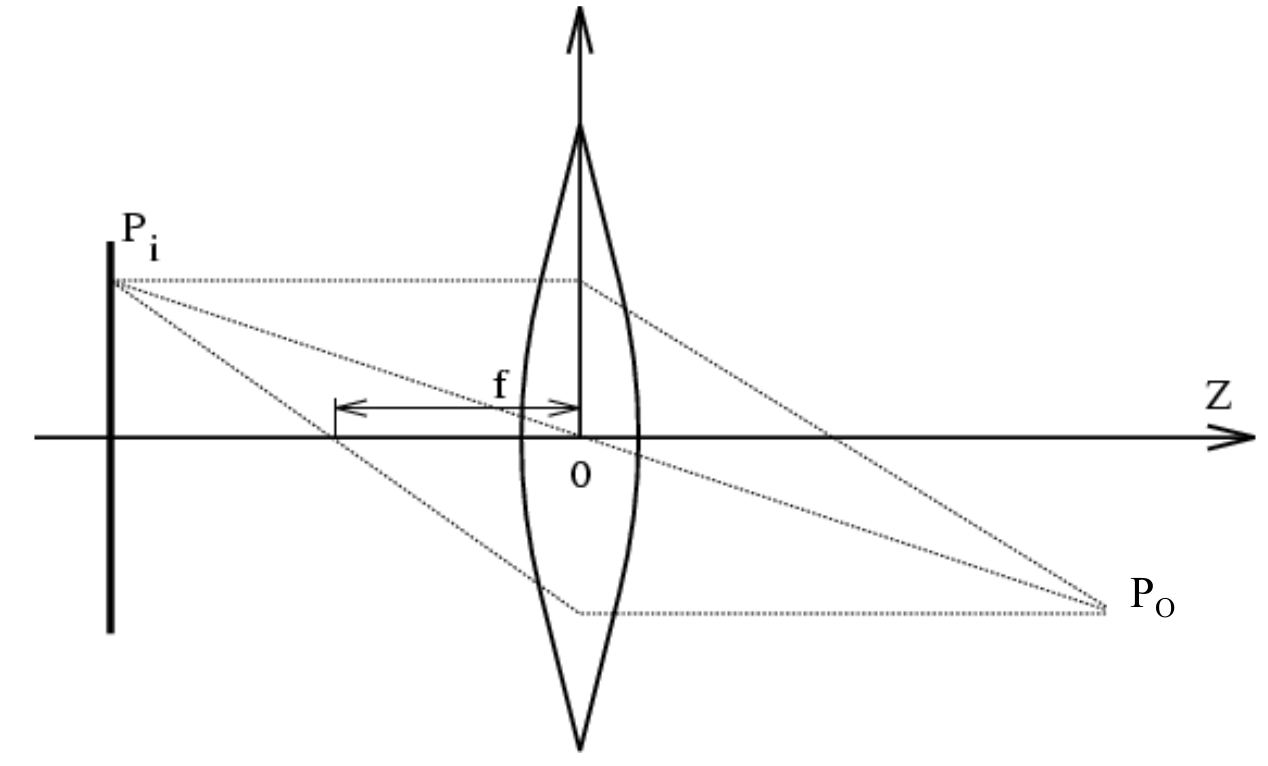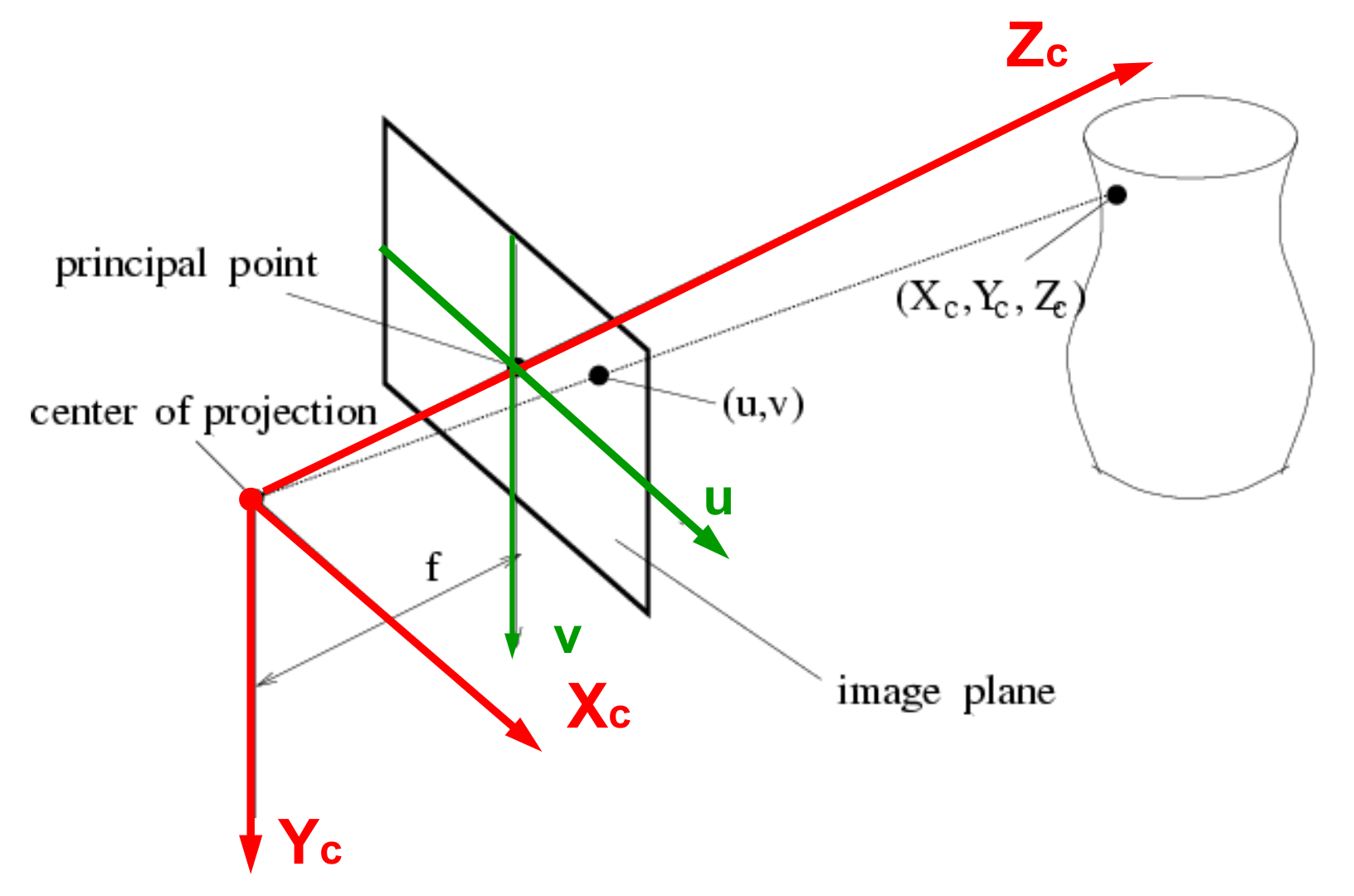# IACV lecture 1: light, lenses and cameras

This is a summary of the introductory lecture to the coure Image Analysis and Computer Vision which I took at ETH in the autumn semester 2018.

## Interaction of light and matter

Interactions of light and matter be divided into three main types (plus diffraction).

Phenomenon Example
Absorption Blue water
Scattering Blue sky, red sunset
Reflection Coloured ink
Refraction Dispersion by a prism, pencil in water
Diffraction Single-slit experiment

Scattering can in turn be divided into three kinds, depending on the relative sized of the wavelength of the light and the size of the particles it is scattering off of:

1. Rayleigh scattering occurs with small particles and is strongly wavelength-dependent.
2. Mie scattering occurs with particles of comparable size to the wavelength, and is mildly wavelength-dependant
3. Non-selective scattering occurs with large particles, and is wavelength-independent.

Reflection can be divided into mirror, diffuse and mixed reflection.

1. Mirror reflection occurs when the angle of reflection is the same as the angle of incidence. The amount of light reflected (reflectance) depends on the angle of incidence and is different for dielectric materials (generally low reflectance except at angles of incidence close to $90\degree$, can polarize light at the Brewster angle) and conductors (generally high reflectance, little effect on polarization).
2. Diffuse (or lambertian) reflection reflects in all directions, independent of incidence angle. It also doesn’t preserve polarization.
3. In the real world, materials always reflect as a mix of reflection and diffusion.Refraction occurs when light changes transmission medium and approximately follows Snell’s law:

$$n_1\sin\theta_1 = n_2\sin\theta_2$$

This is only approximate, since refraction also depends on the wavelength (most apparent in a dispersive prism).

Absorbtion is a dissipation of light of specific wavelengths, depending on the properties of the medium.

## Acquisition of images

Two parts: illumination and cameras.

### 1. Illumination1. Back-lighting
2. Directional lighting
3. Diffuse lighting
4. Polarized lighting
5. Coloured lighting
6. Structured lighting
7. Stroboscopic lighting

### 2. Camera modelsIn the pinhole camera model, light is only allowed to pass through a tiny hole placed at the origin (the hole is large enough that effects of diffraction can be ignored). At a distance $f$ behind the origin, parallel to the $XY$ plane, is the camera sensor array. A point $P_o(x_o,y_o,z_o)$ is projected on the image at $P_i(x_i,y_i,-f)$. This is called perspective projection. Using similar triangles we find:

$$\frac{x_i}{x_o} = \frac{y_i}{y_o} = -\frac{f}{z_o} = -m$$

$m$ is called linear magnification.The issue with a pinhole camera is that since the hole is so small, barely any light comes through and we get a very faint image. We could make the hole bigger, but it would blur the image. The solution is to use a lens.If we make the following assumptions:

1. The lens surfaces are spherical
2. Incoming light rays make small angles with the optical axis ($Z$-axis)
3. The lens thickness is small compared to its radii
4. The refractive index is the same for the media on both sides of the lens

then we can formulate the thin lens equation:

$$\frac{1}{|z_o|} + \frac{1}{|z_i|} = \frac{1}{f}$$

Our lens focuses all the light from a point to the same pixel in our image. This is, however, no free lunch: since the distance between the lens and the sensor ($z_i$) is fixed, the focusing effect only works for objects that are at a specific distance ($z_o$) from the lens. Points closer or further away will be blurred into circles. But how much blur is acceptable? If a pixel in our sensor has size $b$, then if a point forms a circle of radius $\le b$ on our image, we won’t notice a thing. The closest and farthest points where this holds ($P_o^+$ and $P_o^-$ in the image below) define the depth of field.We can show that the following holds (a similar expression can be found for $z_o^+$):

$$\Delta z_o^- = z_o -z_o^- = \frac{z_o(z_o-f)}{z_o + f\frac{d}{b}-f}$$

In other words, an increase in the lens diameter $d$ will cause a reduction of the depth-of-field, and objects further away will be easier to get in focus (larger depth-of-field).

### 3. Aberrations

When an image doesn’t match the above models, we call it an aberration.
There are two main types of aberrations: geometrical and chromatic.

Geometrical aberrations are small for paraxial rays (nearly parallel to the optical axis), but become more pronounced for strongly oblique light rays. They are:

• Spherical aberration: rays parallel to the axis do not converge on the same point. Usually outer are of the lens has smaller focal lengths.
• Radial distortion: variable magnification for different angles of inclination. This can result in bloating the central area of the image (barrel) or shrinking it (pincushion). It can typically be reversed if certain parameters are known (or inferred by looking e.g. at lines that sould be straight).
• Coma
• Astigmatism

Chromatic aberrations are due to the fact that the refraction angle of light also depends on its wavelength, so rays will be focused into different points by the lens. This can be mitigated (achromatization) using composite lenses made from different materials.

### 4. Sensor types

Two main types of camera sensors: CCD (Charge-Coupled Device) and CMOS (Complementary Metal-Oxide Semiconductor).

In CCD sensors, the image is acquired instantaneously in all pixels, and then transferred to memory. This prevents rolling-shutter artefacts but causes slower frame-rates. CCD pixels are also generally more sensitive to light.

In CMOS sensors, each pixel has its own amplifier, which produces more noise but can be used to make “smart” pixels. These pixels are also typically less sensitive but much cheaper. We also can get artefacts due to the rolling shutter. CMOS has effectively taken over the market of digital photography, relegating CCD to niche applications.

### 5. Colour cameras

In colour cameras we want to separate light into three components: red, green, blue. This allows us to record the intensities of these three colours, and reconstruct the original colour of the image (at least as far as our monkey-brains are concerned).

Prism Filer Mosaic Filer Wheel
#sensors 3 1 1
Resolution High Average Good
Cost High Low Average
Frame rate High Low Low
Artefacts Low Aliasing Motion
Bands 3 3 3 or more
High-end cameras Low-end cameras Scientific applications

## Geometric models for camera projection### Perspective projection

Let us introduce a coordinate system where the origin is at the centre of the camera lens and the $Z$-axis is along the optical axis. The image sensor would be parallel to the $XY$ plane at $Z = -f$. However, the image gets reversed by the lens – and when we display it, we “un-reverse” it. It is therefore more convenient to define our image plane at $Z = f$ instead – in front of the camera.
In this coordinate system a point in space $P_c$ is described by the coordinates $(X_c, Y_c, Z_c)$, with subscript $c$ to indicate that this is the camera’s frame of reference. A point on the image plane can be identified with $u,v$ coordinates. By looking at similar triangles we immediately see:
$$u = f\frac{X_c}{Z_c}, \quad v = f\frac{Y_c}{Z_c}$$

### Pseudo-orthographic projection

Imagine standing really far away from your subject and zooming in: the differences in distance from different parts of the subject now become negligible. So by an approximation, we can assume that the distance $Z_c$ is constant in every point of the image. So we get the projection:
$$u = k\cdot X_c, \quad v = k\cdot Y_c$$
with $k = \frac{f}{Z_c}$. This is effectively just a scaling. This model is just an approximation, but can come in handy at times, since it can make certain calculations much simpler.

### Projection matrices and calibration

Let’s make one further generalization to perspective projection. In general, we will have a fixed world frame in which both our camera and our subjects are placed. This means that we can represent the position of our camera with the position $C$ of our centre of projection and a rotation matrix $R$:

$$C = \begin{pmatrix} c_1 \ c_2 \ c_3\end{pmatrix}, \quad R = \begin{pmatrix} r_{11} & r_{12} & r_{13} \ r_{21} & r_{22} & r_{23} \ r_{31} & r_{32} & r_{33}\end{pmatrix}$$

Our image projection becomes:
$$u = f\frac{r_{11}(X-c_1) + r_{12}(Y-c_2) + r_{13}(Z-c_3)}{r_{31}(X-c_1) + r_{32}(Y-c_2) + r_{33}(Z-c_3)},$$
$$v = f\frac{r_{21}(X-c_1) + r_{22}(Y-c_2) + r_{23}(Z-c_3)}{r_{31}(X-c_1) + r_{32}(Y-c_2) + r_{33}(Z-c_3)}.$$

Images, however, are not continuous. They are quantized into picture elements, or pixels. This suggests a further projection of our image coordinates to pixel coordinates $(x,y)$:

$$x = k_xu + sv + x_0, \quad y = k_yv + y_0,$$

where:

• $(x_0,y_0)$ are the pixel coordinates of the principal point,
• $k_x$ is one over the width of one pixel,
• $k_y$ is one over the height of one pixel,
• $s$ indicates the skewness of the pixels – effectively, how far away from being rectangular they are. $s = 0$ means rectangular pixels.

$k_x$, $k_y$, $s$, $x_0$ and $y_0$ are referred to as internal camera parameters: when they are known, the camera is said to be internally calibrated. This effectively means we can convert $(x,y)$ pixel coordinates to $(u,v)$ metric coordinates. If we then want to reconstruct the world coordinates of the projected point, we need to know the position $C$ and the orientation $R$ of the camera. These are known as external camera parameters and the camera will then be externally calibrated. If we know both sets of parameters, our camera will be fully calibrated.

We can rewrite the above projections in matrix format:

$$\rho\begin{pmatrix} u \ v \ 1\end{pmatrix} = \begin{pmatrix} fr_{11} & fr_{12} & fr_{13} \ fr_{21} & fr_{22} &f r_{23} \ r_{31} & r_{32} & r_{33} \end{pmatrix} \begin{pmatrix} X-C_1 \ Y-C_2 \ Z-C_3 \end{pmatrix}$$

$$\mathbf p \coloneqq \begin{pmatrix} x \ y \ 1\end{pmatrix} = \begin{pmatrix} k_x & s & x_0\ 0 & k_y & y_0\ 0 & 0 & 1 \end{pmatrix}\begin{pmatrix} u \ v \ 1\end{pmatrix}$$

for some non-zero $\rho\in\mathbb R$. So defining the calibration matrix:

$$K = \begin{pmatrix} k_x & s & x_0\ 0 & k_y & y_0\ 0 & 0 & 1 \end{pmatrix}\begin{pmatrix} f & 0 & 0\ 0 & f & 0\ 0 & 0 & 1 \end{pmatrix} = \begin{pmatrix} fk_x & fs & x_0\ 0 & fk_y & y_0\ 0 & 0 & 1 \end{pmatrix}$$

we get:

$$\boxed{\rho\mathbf p = KR(\mathbf{P-C})}$$